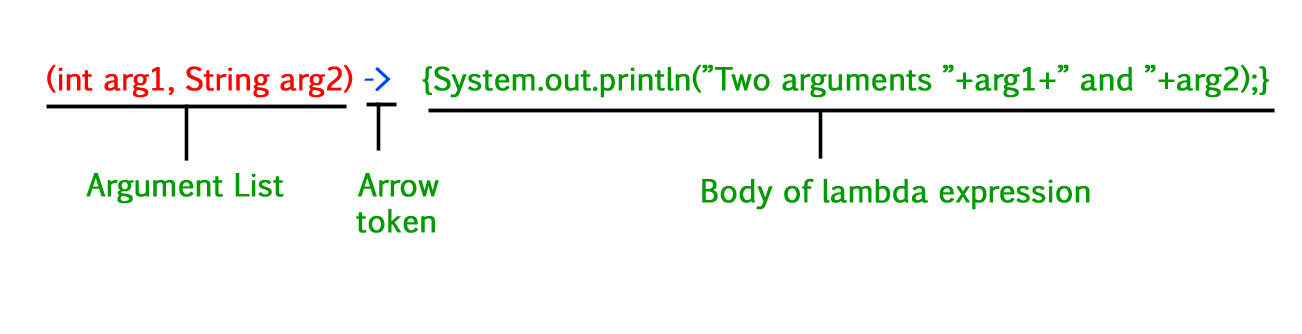# Java – Lambda Expressions Parameters

• Difficulty Level : Basic
• Last Updated : 24 Jun, 2022

Lambda Expressions are anonymous functions. These functions do not need a name or a class to be used. Lambda expressions are added in Java 8. Lambda expressions basically express instances of functional interfaces An interface with a single abstract method is called a functional interface. One example is java.lang.Runnable.

Lambda expressions implement only one abstract function and therefore implement functional interfaces. Predicate interface is an example of a functional interface that has only one abstract method called test().

Illustration:

```interface Predicate
{
......
abstract boolean test(T t)
}```

The above is a functional interface that has one abstract method test receiving only one parameter of type T and returns a boolean value. This method is a generic method that takes a type parameter. This interface can be implemented anywhere in a program using a lambda expression instead of creating classes with multiple functions. For eg, to implement a runnable interface used only for multithreading one needs to implement only a run() method. Then there is the comparable interface which can be implemented using compare() method.

Important points:• The body of a lambda expression can contain zero, one, or more statements.
• When there is a single statement curly brackets are not mandatory and the return type of the anonymous function is the same as that of the body expression.
• When there is more than one statement, then these must be enclosed in curly brackets (a code block) and the return type of the anonymous function is the same as the type of the value returned within the code block, or void if nothing is returned.

These are for singleline lambda expressions having void return type.

Type 1: No Parameter.

Syntax:

`() -> System.out.println("Hello");`

It takes interface of the following form:

```interface Test1
{
void print()
}```

Type 2: Single Parameter.

Syntax:

`(p) -> System.out.println(p);`

It is not mandatory to use parentheses if the type of that variable can be inferred from the context

It takes interface of the following form:

```interface Test2
{
void print(Integer p)
}```

The type and return type of the lambdas are automatically inferred.

Type 3: Multi parameters

`(p1, p2) -> System.out.println(p1 + " " + p2);`

It is not mandatory to use parentheses if the type of that variable can be inferred from the context

It takes interface of the following form:

```interface Test3
{
void print(Integer p1, Integer p2)
}```

The type and return type of the lambdas are automatically inferred.

Now, we are done with discussing out the theoretical concept, now let us come up with the implementation part. So here primarily we will be discussing out the codes for the above three types as discussed above:

Note: forEach() method is of Iterable interface that is used to iterate through a collection. Here it takes an argument of Consumer type interface. This is a functional interface having only one abstract method called accept(). Since it is a functional interface, a lambda expression can be passed.

Hence, if we do conclude out the above

Example 1: Lambda expression with no parameters

## Java

 `// Java code to illustrate lambda expression` `// without parameters`   `// functional interface` `// without parameters` `interface` `Test1 {` `    ``void` `print();` `}`   `class` `GfG {` `    ``// functional interface parameter is passed` `    ``static` `void` `fun(Test1 t) { t.print(); }` `    ``public` `static` `void` `main(String[] args)` `    ``{` `        ``// lambda expression is passed` `        ``// without parameter to functional interface t` `        ``fun(() -> System.out.println(``"Hello"``));` `    ``}` `}`

Output

`Hello`

Example 2: Type 2 Lambda expression with a single parameter

## Java

 `// Java code to illustrate lambda expression` `// with single parameter`   `// functional interface` `// with one parameter of Integer type` `interface` `Test2 {` `    ``// The void type and the Integer type` `    ``// is automatically inferred from here` `    ``// and assigned to the lambda expression` `    ``void` `print(Integer p);` `}`   `class` `GfG {` `    ``// takes lambda expression and a variable of` `    ``// Integer type as arguments` `    ``static` `void` `fun(Test2 t, Integer p)` `    ``{` `        ``// calls the print function` `        ``t.print(p);` `    ``}` `    ``public` `static` `void` `main(String[] args)` `    ``{` `        ``// lambda expression is passed` `        ``// with a single parameter` `        ``// lambda expression is mapped to the` `        ``// single argument abstract function in the` `        ``// functional interface Test2` `        ``fun(p -> System.out.println(p), ``10``);` `    ``}` `}`

Output

`10`

Example 3: Type 3 Lambda expression with multi parameters

## Java

 `// Java code to illustrate lambda expression` `// with multi parameters`   `// functional interface Test3` `// with 2 parameter of Integer type` `interface` `Test3 {` `    ``// The void type and the Integer type` `    ``// is automatically inferred from here` `    ``// and assigned to the lambda expression` `    ``void` `print(Integer p1, Integer p2);` `}`   `class` `GfG {` `    ``// takes parameter of Test3 type followed` `    ``// by 2 integer parameters p1 and p2` `    ``static` `void` `fun(Test3 t, Integer p1, Integer p2)` `    ``{` `        ``// calls the print function` `        ``t.print(p1, p2);` `    ``}` `    ``public` `static` `void` `main(String[] args)` `    ``{` `        ``// lambda expression is passed` `        ``// with two parameters` `        ``// lambda expression is mapped to the` `        ``// double argument abstract function in the` `        ``// functional interface Test3` `        ``fun((p1, p2)` `                ``-> System.out.println(p1 + ``" "` `+ p2),` `            ``10``, ``20``);` `    ``}` `}`

Output

`10 20`

Example 4:Lambda expression with two parameters

## Java

 `// Demonstrate a lambda expression that takes two parameters`   `interface` `NumericTest2{` `   ``boolean` `test(``int` `n, ``int` `d);` `}`   `class` `GFG{` `public` `static` `void` `main(String args[]){`   `  ``// The labda expression here determines if one number is the factor of another` `  ``NumericTest2 isFactor= (n,d) -> (n%d)==``0``;` `  ``if``(isFactor.test(``10``,``2``))` `    ``System.out.println(``"2 is the factor of 10"``);` `  `  `  ``if``(!isFactor.test(``10``,``3``))` `    ``System.out.println(``"3 is not a factor of 10"``);` `  `  `}` `}`

Output

```2 is the factor of 10
3 is not a factor of 10```

My Personal Notes arrow_drop_up
Recommended Articles
Page :